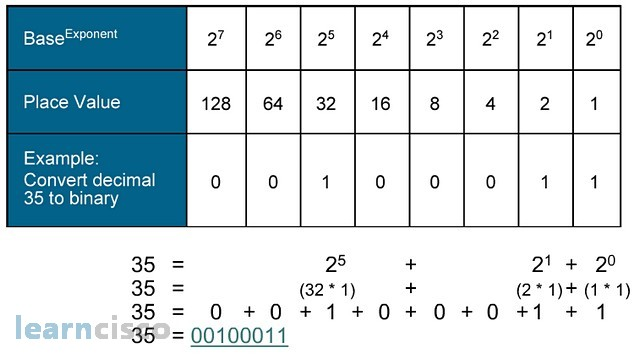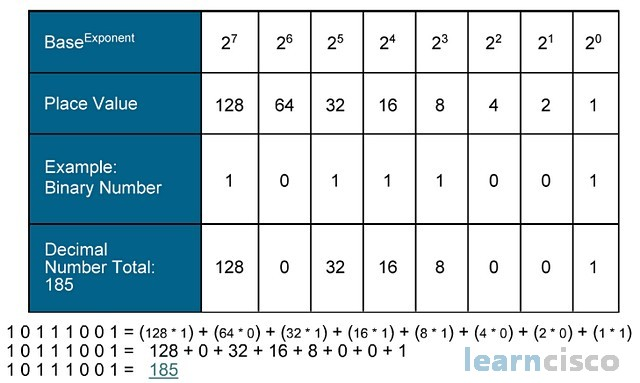ICND1 100-105

# Understanding Binary Basics

IP addresses are nothing more than a string of 32 binary digits. In order to understand their operations, this lesson describes a review of the binary system. We will start by comparing that system with the decimal system and explain how the number 2 is a building block for the rest of the process. You will actually get the chance to practice converting a decimal number to a binary number and vice versa.

## Decimal vs. Binary Numbers

If binary numbers are so important in IP addressing and subnetting, let us take a deeper look at their structure and values. We would also need to take a look at how to convert them into decimal numbers because we human beings are not that smart and we do not want to deal with binary numbers. We want to deal with something we are used to, decimal numbers, that is, in fact, perhaps the best way to understand binary numbers to compare them to something we know like decimal numbers. So the first philosophical question of the day is what is a decimal number? We know that because we work with them on an every day basis. Our base number is 10 and a decimal number is nothing more than a string of digits that go from 0 through 9. Now in decimal numbers, we know that it is location, what makes sense out of the digit within the number; in other words, we know what the first digit in the first location or place means. We need that the value is a number itself; it is less than 10. We also know that the second location or place is the tens, and so we know that the actual value of that digit is the digit multiplied by 10 and so on, we know the third location is hundreds, and the fourth is thousands, and the fifth is tens of thousands, and so on. All of those tens, hundreds, thousands, etc., are nothing more than powers of 10.

Decimal Binary Decimal Binary 0 0000 9 1001 1 0001 10 1010 2 0010 11 1011 3 0011 12 1100 4 0100 13 1101 5 0101 14 1110 6 0110 15 1111 7 0111 16 10000 8 1000 17 10001

Well, a binary number is exactly the same but at this time; the base is 2 and so our possible digits are 0 and 1. This is what computers use, because it is easier to implement the computer if the intelligence is reduced to two numbers. We could build them with a base 10, but they would be awfully expensive. In any case, building binary numbers follows the same procedure or logic than building a decimal number. A binary number will be nothing more than a string of digits, where each digit has to be 0 or 1 and it is the location of the digit within the number that makes sense out of it. All locations also represent powers of the base, but this time the base is 2, so locations will represent powers of 2.

## Decimal to Binary - Theory

It is quite strange though to look at a string of binary digits and quickly tell what the value is. Well, I am there to say that it is just a matter of getting used to it. We are used to decimal numbers and we know how to calculate the total value of a number, at least estimate it or at least have an idea of what it is. We do these things in our minds, but you can tell if our number is that, one of those digits. Then we quickly go about our business and know that first digit is 9, in this case, the second digit is going to be in the 10s and so because it is a 2, it represents the value of 20, the third digit is 8, but it is in the third location and so it represents 100s, which means it is 800 and on and on it goes. Now we probably do not think of too much about this, but all those values that are associated with the places or locations are nothing more than powers of 10.

### Base-10 Decimal Conversion - ex. 63204829

MSBLSB
Baseexponent 107 106 105 104 103 102 101 100
Column Value 6 3 2 0 4 8 2 9
Decimal Weight 10000000 1000000 100000 10000 1000 100 10 1
Column Weight 60000000 3000000 200000 0 4000 800 20 9

60000000 + 3000000 + 200000 + 0 + 4000 + 800 + 20 + 9 = 63204829

Well again, it is a matter of getting used to it. A binary conversion or calculating the value of a binary number is going to follow exactly the same process. This time we will have numbers that look like that, 1s and 0s. Now we apply the same principles and you can derive the fact that each digit will have to be multiplied this time by the power of 2, because our base is 2, so that first one is multiplied by 1, which is 2 to the 0 power, the second digit, the 0, has to be multiplied by 2, which is 2 to the first power, third digit multiplied by 2 to the second power, fourth digit multiplied by 2 to the third power, and so on. We probably need to think in terms of the least significant bit and the most significant bit, which has the same logic in either binary or decimal notations.

### Base-2 Binary Conversion - ex. 1110100 (233)

MSBLSB
Baseexponent 27 26 25 24 23 22 21 20
Column Value 1 1 1 0 1 0 0 1
Decimal Weight 128 64 32 16 8 4 2 1
Column Weight 128 64 32 0 8 0 0 1

128 + 64 + 32 + 0 + 8 + 0 + 0 + 1 = 233

The powers of 2 start at the least significant bit and the exact location or place of the digit or actual power of 2 that we need to multiply by. Starting with 0, we have eight locations in this example and it make sense, because eight locations is 8 bits and 8 bits, it is a byte. Remember we group together bits and bytes, separated by dots and come up with IP addresses, so the actual value of that binary number is decimal 233 in this example.

## Powers of 2

So our main problem, which is to look at a binary number and quickly calculate its decimal value, because we want to see and understand IP addresses, it is reduced to knowing the powers of 2, because we know that location is important and the location will give us the power of 2 with that we need to use in our calculations. Well if we consider the byte again, 8 bits, then all we have to do is probably memorize eight values: first power 2 is 2 elevated to the 0 power, which is 1, 2 to the first power is 2, 2 to the second power is 4, and so on and so forth until you get to all those values. Think about it. It is not so difficult: 1, 2, 4, 8, 16, 32, 64, and 128; there you go.

Power of 2CalculationValue
20 1
21 2 2
22 2 * 2 4
23 2 * 2 * 2 8
24 2 * 2 * 2 * 2 16
25 2 * 2 * 2 * 2 * 2
32
26 2 * 2 * 2 * 2 * 2 * 2 64
27 2 * 2 * 2 * 2 * 2 * 2 * 2
128

## Decimal to Binary Conversion Example

In IP addressing, especially in subnetting, it is sometimes useful to convert decimal numbers to binary numbers. This is especially true in subnetting, which is a process we will review in our next lesson. Well, this is the way to do it. We know that a decimal value is going to be a string of binary digits in a binary number. Each digit is going to have a certain decimal value according to its location. So, for example, 1 in the seventh location, with the understanding that the seventh location is actually the eighth place, if we count from 0 to 7, then a number 1 on that location is going to represent the number of 128, and number 1 in the third location again from 0 through 7 is going to represent a decimal 8. So with our powers of 2 in mind, all we have to do is find the first power of 2 that fits into the decimal number we want to convert. We set that place or location to a binary 1 and then subtract it from the original decimal number.We then repeat the process until the result of our subtractions is 0; let us take a look at this example, with this in mind, we say 35, what is the first power of 2 that fits into the 35? So obviously 32; 128 and 64 do not fit in the decimal number and so they will have a binary value of 0 on that location or place, so the first one is 32. We put a 1 in that location and subtract 32 from 35, the resulting decimal number is 3. Let us repeat the process; what is the next best power or 2 that fits into 3? Well 16, 8, and 4 do not fit in 3. They are all greater than 3 and so they will all have a 0 in that location, so the next best power of 2 is number 2, so we put another 1 in that location and subtract, 3 minus 2 is 1 next best power of 2 is that 1 and that means that location gets a 1, subtract it get a 0, and we are done. It means decimal 35 is 00100011.

## Binary to Decimal Conversion Example

Binary to decimal conversions are even easier; all we have to do is apply the same process that took us to the understanding of binary numbers. The process goes like this: we take the digits in our binary number and multiply them by the power of 2 that corresponds to their location, starting with location 0 on the right or least significant bit and moving to the left until we hit location 7, which is the most significant bit. We then add up all of the resulting numbers together, so if that is our binary number in the example. Then all we have to do is add up all those powers of 2; the number 1 means we are going to multiply 1 by the corresponding power of 2, which is also 1 there.The 0 there means that we multiply 0 by the power of 2. The end result is going to be that nothing is added; same thing goes for the third location there. The fourth location is a 1, so multiply that by the corresponding power of 2, which is in this case 2 to the third power, and we add it up to the total. To continue doing this, up to the most significant bit, then you will have a series of decimal numbers that need to be added together, and that is going to result in a decimal number again. So that binary number is decimal 185.